# Relative Atomic Mass

## Key Stage 3

### Meaning

The Atomic Mass or mass number is the number of nucleons (protons + neutrons) in an atom.

Two atoms of the same element may have the same Atomic Number but a different Atomic Mass depending on the number of neutrons in the nucleus. Elements with different mass numbers are called isotopes.
The atomic mass is not affected by the number of electrons.
Only the particles in the nucleus affect the atomic mass.

### Examples

 Hydrogen Helium Lithium Beryllium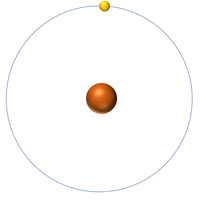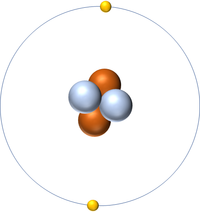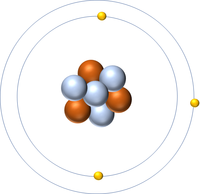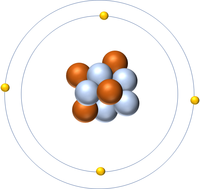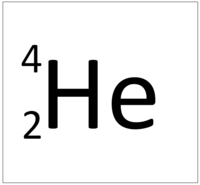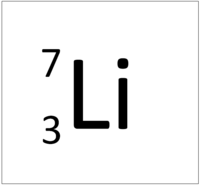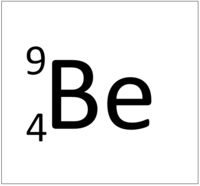Hydrogen has one nucleon so it has an atomic mass of 1. Helium has four nucleons so it has an atomic mass of 4. Lithium has seven nucleons so it has an atomic mass of 7. Beryllium has nine nucleons so it has an atomic mass of 9.

## Key Stage 4

### Meaning

The relative atomic mass or mass number of an element is the number of nucleons (protons + neutrons) in an atom.

The relative atomic mass in grams is also the mass of one mole or 6.02x1023 atoms of the element.

 Hydrogen Deuterium Tritium Boron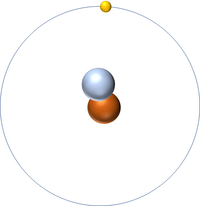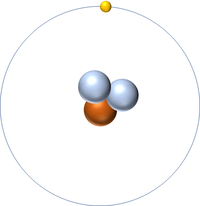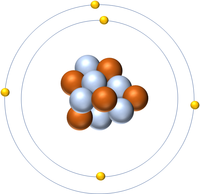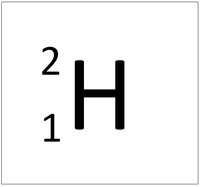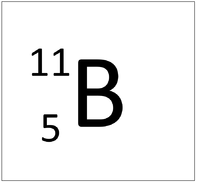Hydrogen has one nucleon so it has an atomic mass of 1 and 6.02x1023 (or 1 mole of) atoms of Hydrogen have a mass of 1g. Deuterium has two nucleons so it has an atomic mass of 2 and 6.02x1023 (or 1 mole of) atoms of Deuterium have a mass of 2g. Tritium has three nucleons so it has an atomic mass of 3 and 6.02x1023 (or 1 mole of) atoms of Tritium have a mass of 3g. Boron has eleven nucleons so it has an atomic mass of 11 and 6.02x1023 (or 1 mole of) atoms of Boron have a mass of 11g.